## Solution to 1986 Problem 18

 Presumably, the Schrodinger equation" in the problem statement refers to the time-independent Schrodinger equation in one dimension, which is given by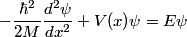\begin{align*}-\frac{\hbar^2}{2 M} \frac{d^2 \psi}{dx^2} + V(x) \psi = E \psi\end{align*}First, we compute the second derivative of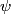$\psi$.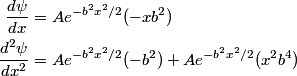\begin{align*}\frac{d \psi}{dx} &= A e^{-b^2 x^2/2} (- xb^2) \\\frac{d^2 \psi}{dx^2} &= A e^{-b^2 x^2/2} (- b^2) + A ...We plug this into the Schrodinger equation to find that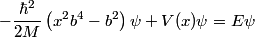\begin{align*}-\frac{\hbar^2}{2 M} \left(x^2 b^4 - b^2 \right) \psi + V(x) \psi = E \psi\end{align*}Now evaluate the above equation at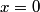$x = 0$ to find that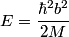\begin{align*}E = \frac{\hbar^2 b^2}{2M}\end{align*}Now plug this back into the Schrodinger equation.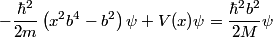\begin{align*}-\frac{\hbar^2}{2 m} \left(x^2 b^4 - b^2 \right) \psi + V(x) \psi = \frac{\hbar^2 b^2}{2M} \psi\end{align*}Divide through by$\psi$ to find that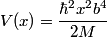\begin{align*}V(x) = \frac{\hbar^2 x^2 b^4}{2M}\end{align*}Hence, answer (B) is correct.